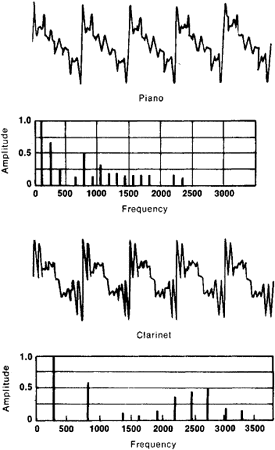Sound, Analysis of

The following article is from The Great Soviet Encyclopedia (1979). It might be outdated or ideologically biased.

Sound, Analysis of

the expansion of a complex sound process into a series of simple vibrations. Two types of sound analysis are used—frequency and time.

In frequency sound analysis, the acoustic signal is represented as the sum of harmonic components, which are characterized by their frequency, phase, and amplitude. Frequency sound analysis makes it possible to find the frequency distribution of the components’ amplitudes (see Figure 1) —the so-called frequency-amplitude spectra—and less often the frequency distribution of the components’ phases (the phase-frequency spectra). If the spectrum of a noise, such as the noise of a motor vehicle, is known—that is, if the frequencies and amplitudes of its harmonics are known—it is possible to design a silencer. Knowledge of the spectra of speech and musical signals makes possible the proper design of the frequency-response curve of transmission channels, to provide the required quality of reproduction. To calculate the fatigue strength of rocket components and to prevent their failure when exposed to engine noise, the sound spectrum of the engines must be known.Figure 1. Shape of oscillations and frequency-amplitude spectra of sounds from a piano (frequency 128 Hz) and a clarinet (275 Hz)

In a time sound analysis the signal is represented as the sum of short pulses that are characterized by the time of their appearance and by their amplitude. The methods of time sound analysis are the basis for the principle of operation of sonars and depth finders. The determination of the time of arrival of the pulses makes possible the determination of the distance to an object or the depth of a body of water. The nature of an object or the bottom may be judged from the amplitude of the reflected signal. In practice it often becomes necessary to have a characteristic that gives a general picture of a signal’s time variation without expanding it into harmonic or pulse components. The so-called correlation function, which is defined as the time average product of the multiplication of the analyzed signal by its delayed version (autocorrelation) or by the delayed version of a second analyzed signal (cross-correlation), is often used for this purpose. Methods of correlation analysis are used to solve such problems as predicting the nature of a change in a process with time, the separation of weak acoustic signals from background noise, and the measurement of the distortion of broadcast signals during transmission through electroacoustic systems. Many physical characteristics of acoustic processes, systems, and sound fields that are of practical interest may be found from correlation functions.

REFERENCES

Blinova, L. P., A. E. Kolesnikov, and L. B. Langans. Akusticheskie izmereniia. Moscow, 1971.
Kharkevich, A. A. Spektry i analiz, 4th ed. Moscow, 1962.

N. N. PISAREVSKII